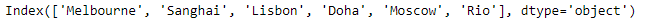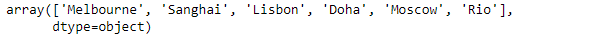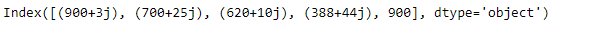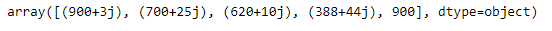Skip to content
Related Articles
Python | Pandas Index.values
• Last Updated : 20 Feb, 2019

Pandas Index is an immutable ndarray implementing an ordered, sliceable set. It is the basic object which stores the axis labels for all pandas objects.

Pandas` Index.values` attribute return an array representing the data in the given Index object.

Syntax: Index.values

Parameter : None

Returns : an array

Example #1: Use `Index.values` attribute to return an array representing the data in the given Index object.

 `# importing pandas as pd``import` `pandas as pd`` ` `# Creating the index``idx ``=` `pd.Index([``'Melbourne'``, ``'Sanghai'``, ``'Lisbon'``, ``'Doha'``, ``'Moscow'``, ``'Rio'``])`` ` `# Print the index``print``(idx)`

Output :Now we will use `Index.values` attribute to return an array representing the data in the given Index object.

 `# return an array``result ``=` `idx.values`` ` `# Print the result``print``(result)`

Output :As we can see in the output, the `Index.values` attribute has successfully returned an array representing the data of the given Index object.

Example #2 : Use `Index.values` attribute to return an array representing the data in the given Index object.

 `# importing pandas as pd``import` `pandas as pd`` ` `# Creating the index``idx ``=` `pd.Index([``900` `+` `3j``, ``700` `+` `25j``, ``620` `+` `10j``, ``388` `+` `44j``, ``900``])`` ` `# Print the index``print``(idx)`

Output :Now we will use `Index.values` attribute to return an array representing the data in the given Index object.

 `# return an array``result ``=` `idx.values`` ` `# Print the result``print``(result)`

Output :As we can see in the output, the `Index.values` attribute has successfully returned an array representing the data of the given Index object.

Attention geek! Strengthen your foundations with the Python Programming Foundation Course and learn the basics.

To begin with, your interview preparations Enhance your Data Structures concepts with the Python DS Course. And to begin with your Machine Learning Journey, join the Machine Learning – Basic Level Course

My Personal Notes arrow_drop_up